Math Concepts

# How to get started with Geometrical Shapes?

0

Table of Contents

 1 Introduction 2 What are Geometrical Shapes? 3 Types of Lines 4 Different Types of Shapes and their Names 5 Types of 2D Shapes, Names & their Definition 6 Types of 3D Shapes, Names & their Definition 7 Example Problems 8 Frequently Asked Questions (FAQs) on Geometrical Shapes 9 External References

28 January 2021

Reading time: 4 minutes

What is geometry?

Geometry is one of the oldest branches of Mathematics and the term is derived from Greek.  The word “Geo” means “Earth” and “Metry” means “Measurement.” Therefore, Geometry means “Earth Measurement.”

Geometry is the fun-based branch of Mathematics that deals with the study of shapes, sizes, relative positions of objects, dimensions, and properties of space.

Geometry is the rules of all mental investigation. Cuemath guides the students to visualize this branch of Mathematics by helping them to understand the shape and size of the individual objects, the spatial relationships among the objects, and the properties surrounding the space.

## What are Geometrical Shapes?

Geometrical shapes are the shapes which we see in our daily life.  Geometrical Shapes are defined as a figure or area enclosed by a boundary that is created by combining the specific number of curves, points, and lines.

Some of the examples of Geometrical Shapes are Triangle, Square, Rectangle, etc. Download the PDF to understand Geometrical Shapes in detail.

 📥 Geometrical Shapes Download

Also, read

## Types of Lines

Before we learn about different Geometrical Shapes, we have to know the different types of lines that are used in constructing Geometric Shapes.
The two major types of Lines are:

1. ### Straight Line

A line that is thin, one dimensional, a zero-width object that extends on both sides of the infinity is called a Straight Line.

The Straight Line is of three types. They are:

• Slanting Line
• Horizontal Line (Sleeping Line)
• Vertical Line (Standing Line)
1. ### Curved Line

A line in which the point does not move in one direction is called a Curved Line.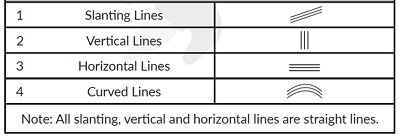## Different Types of Shapes and their Names

Geometry is all about shapes and their properties. After we have learned about the different types of lines, we will learn about different shapes, their names, and their properties.

In Geometry, shapes are the figures which have boundary lines, angles, and surfaces. The shapes are classified into two types and they are 2D shapes and 3D shapes

1. ### 2D Shapes (Two Dimensional Shapes)or Flat Shapes

The Geometrical Shapes or figures that have only two dimensions i.e., width and length but not thickness is called 2D Shapes or Flat Shapes. Some of the examples are Triangle, Rectangle, Square, Circle, etc.

1. ### 3D Shapes (Three Dimensional Shapes) or Solid Shapes

The Geometrical Shapes or figures that have three dimensions i.e., width, length, and thickness is called 3D Shapes or Solid Shapes. Some of the examples for the solid shapes are Cylinder, Cone, Sphere, Cube, Cuboid, etc.

## Types of 2D Shapes, Names & their Definition

2D Shape Name Shape Definition
Triangle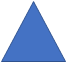A triangle is a plane shape formed by three lines
Square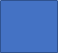A square is a 2D Shape, formed by four lines of equal length.
Rectangle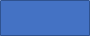A rectangle is a plane shape that is formed by four lines in which the opposite sides are equal and parallel.
Circle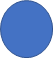A circle is a round-shaped figure with all the points at the same distance from the centre, with no sides and corners.
Oval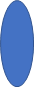An oval is a curved 2D figure with a slightly elongated outline, and it looks similar to an egg, with no sides and corners.

## Types of 3D Shapes, Names & their Definition

3D Shape Name Shape Definition
Cube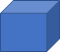A cube is a 3D shape, made up of six equal squares. It is also called a regular hexahedron.
E.g., Rubik’s Cube
Cuboid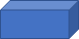A cuboid is a 3D shape that is formed by six equal rectangles.
E.g., Book, Box
Cylinder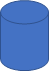A cylinder is a solid shape that has two identical flat ends that are circular with one curved side.
E.g., Pipes, Gas Cylinder
Sphere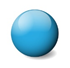A sphere is a solid figure which looks like a ball.
E.g., Football, Globe
Cone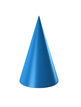A cone is a 3D shape that narrows smoothly from a flat circular base to a point.
E.g. Ice-Cream Cone

## Example Problems

Question 1: What is the shape of the shaded part?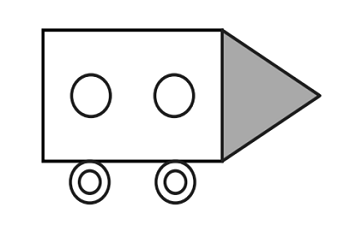(A) Circle

(B) Rectangle

(C) Triangle

(D) Square

 (C) Triangle

Question 2: How many more straight lines than curved lines are there in the given figure?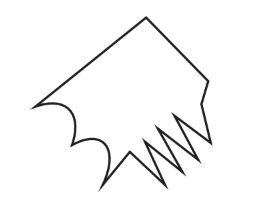(A)  8
(B)  7
(C)  9
(D) 10

 (D) 10

Question 3: How many squares and circles are there in the given figure?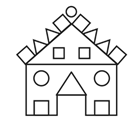(A)  3,4
(B)  8,3
(C)  3,2
(D) 4,3

 (B) 8,3

Question 4: What is the number of slanting lines in the given figure?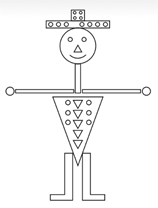(A)  13
(B)  14
(C)  15
(D) 17

 (B) 14

Question 5:  A triangle is a closed plane shape with
(A)  3 Sides
(B)  4 Sides
(C)  5 Sides
(D) 7 Sides

 (A) 3 sides

Question 6: How many cubes are in the below-given figure?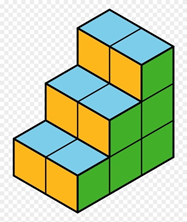(A)  10
(B)  12
(C)  6
(D) 8

 (B) 12

Question 7: Which of the following is a sphere?
(A)  Battery Cell
(B)  Football
(C)  Match Box
(D) Ice Cream

 (B) Football

Question 8: When a ball is pushed forward. Will it roll, or will it slide?
(A)  Roll
(B)  Slide

 (A) Roll

Question 9:  How many sleeping lines are there in the alphabet “E”?
(A)  4
(B)  5
(C)  3
(D) 2

 (C) 3

Question 10: Which of the following statements are true?
(A)  A cuboid has six square faces
(B)  A cube has a curved face
(C)  A cylinder has one curved face and two flat circular faces
(D) A cone has two curved face and two circular faces

 (C) A cylinder has one curved face and two flat circular faces

Question 11: How many sleeping lines, slanting lines, standing lines are there in the given figure.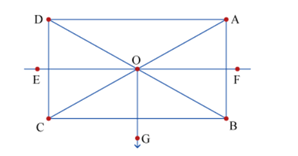(A) 4, 4, 3
(B) 3, 4, 3
(C) 4, 5, 2
(D) 3, 3, 3

 (B)  3, 4, 3

Question 12: Which of the following letters has zero straight lines?
(A)  B
(B)  A
(C)  C
(D) P

 (C) C

## About Cuemath

Cuemath, a student-friendly mathematics and coding platform, conducts regular Online Live Classes for academics and skill-development, and their Mental Math App, on both iOS and Android, is a one-stop solution for kids to develop multiple skills. Understand the Cuemath fee structure and sign up for a free trial.

## What are the basic geometrical shapes?

Triangle, square, rectangle and circle.

## What is the definition of geometric shapes?

Geometric shapes are shapes made out of points and lines including the triangle, square, and circle.

## External References

Related Articles
GIVE YOUR CHILD THE CUEMATH EDGE
Access Personalised Math learning through interactive worksheets, gamified concepts and grade-wise courses
Learn More About Cuemath